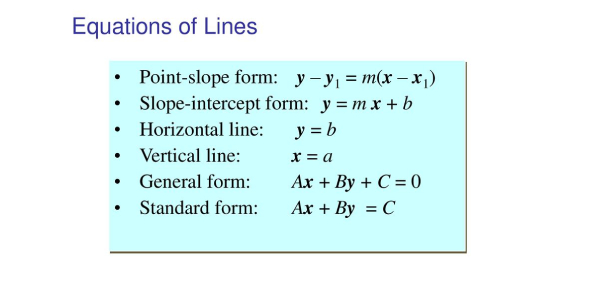# Equations Of Lines Quiz! Math Trivia

12 Questions | Attempts: 2555SettingsWhat do you understand with a linear equation? A linear equation is a calculation whose graph is a line. Equations containing one or two variables can be graphed on any x_y coordinate plane. If a point lies on the equation graph, its coordinates render the equation a true statement. If a coordinate of a point makes an equation a true statement, then the point lies in the equation's graph. You simply must take this excellent quiz.

• 1.
What is the equation of a line with a slope of 8 and a y-intercept of (0, -2)?
• A.

Y = -2x + 8

• B.

Y = 8x - 2

• C.

Y = -16x

• D.

Y = 1/8x - 2

• 2.
What is the slope of the given line: y = 2/3x + 5
• A.

2/3

• B.

5

• 3.
What is the slope of the line with the equation y = -4x + 9?
• A.

9

• B.

1/4

• C.

-4

• D.

1/9

• E.

Cannot be determined

• 4.
What is the y-intercept of the line with equation y = 1/5x + 2?
• A.

(0, 1)

• B.

(0, 5)

• C.

(0, 2)

• D.

(0, -2)

• E.

Cannot be determined

• 5.
What is the y-intercept of the line with equation y = -5x + 6?
• A.

(0, -6)

• B.

(0, 6)

• C.

(0, 5)

• D.

(0, -5)

• E.

Cannot be determined

• 6.
Which equation is parallel to y = 3/4x + 5?
• A.

Y = 4/3x + 1

• B.

Y = -4/3x + 1

• C.

Y = 3/4x + 1

• D.

Y = -3/4x + 1

• E.

Y = 8x + 5

• 7.
Which of the following is an equation of a line perpendicular to y = 3/4x + 5?
• A.

Y = 4/3x + 1

• B.

Y = -4/3x + 1

• C.

Y = 3/4x + 1

• D.

Y = -3/4x + 1

• E.

Y = 8x + 5

• 8.
What is the equation of the line passing through (2, 3) and (4, 5)?
• A.

Y = -x + 1

• B.

Y = x + 1

• C.

Y = 2x + 1

• D.

Y = -2x + 1

• E.

Cannot be determined

• 9.
What is the equation of the line passing through (3, 5) and (-1, -3)?
• A.

Y = -2x + 11

• B.

Y = -2x - 5

• C.

Y = 4x - 7

• D.

Y = 2x - 1

• E.

Y = 2x + 1

• 10.
What is the equation of the line passing through (0, 4) and (3, 6)?
• A.

Y = 2/3x + 4

• B.

Y = -2/3x + 4

• C.

Y = 3/2x + 4

• D.

Y = -3/2x + 1.5

• E.

Cannot be determined

• 11.
What is the equation of the line passing through (4, 6) and (4, -2)?
• A.

X = 4

• B.

Y = 8

• C.

Y = -8

• D.

Cannot be determined

• 12.
What is the equation of the line passing through (3, 5) and (2, 5)?
• A.

X = 1

• B.

X = -1

• C.

Y = 5

• D.

Cannot be determined

## Related TopicsBack to top
×

Wait!
Here's an interesting quiz for you.